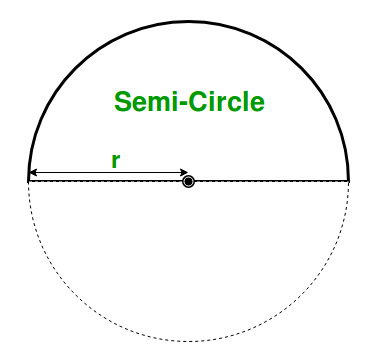# Program to find the Area and Perimeter of a Semicircle

Given the radius of the semicircle as r, the task is to find out the Area and Perimeter of that semicircle.

Examples:

```Input: r = 10
Output: Area = 157.00, Perimeter = 31.4

Input: r = 25
Output: Area =981.250000, Perimeter = 78.500000
```

## Recommended: Please try your approach on {IDE} first, before moving on to the solution.

Approach:
In mathematics, a semicircle is a one-dimensional locus of points that forms half of a circle. The area of a semicircle is half the area of the circle from which it is made. Any diameter of a circle cuts it into two equal semicircles.Area of Semi-Circle = 12 * π *r2
Perimeter of Semi-Circle = π *r

where “r” is the radius of the semicircle.

Below is the implementation of the above approach:

## C++

 `// C++ program to find the ` `// Area and Perimeter of a Semicircle ` ` `  `#include ` `using` `namespace` `std; ` ` `  `// Function for calculating the area ` `float` `area(``float` `r) ` `{ ` `    ``// Formula for finding the area ` `    ``return` `(0.5)*(3.14)*(r * r); ` `} ` ` `  `// Function for calculating the perimeter ` `float` `perimeter(``float` `r) ` `{ ` `    ``// Formula for finding the perimeter ` `    ``return` `(3.14)*(r); ` `} ` ` `  `// driver code ` `int` `main() ` `{ ` ` `  `    ``// Get the radius ` `    ``int` `r = 10; ` ` `  `    ``// Find the area  ` `    ``cout << ``"The Area of Semicircle: "` `         ``<< area(r) << endl; ` ` `  `    ``// Find the perimeter ` `    ``cout << ``"The Perimeter of Semicircle: "` `         ``<< perimeter(r) << endl; ` ` `  `    ``return` `0; ` `} `

## C

 `// C program to find the ` `// Area and Perimeter of a Semicircle ` ` `  `#include ` ` `  `// Function for calculating the area ` `float` `area(``float` `r) ` `{ ` `    ``// Formula for finding the area ` `    ``return` `(0.5)*(3.14)*(r * r); ` `} ` ` `  `// Function for calculating the perimeter ` `float` `perimeter(``float` `r) ` `{ ` `    ``// Formula for finding the perimeter ` `    ``return` `(3.14)*(r); ` `} ` ` `  `// driver code ` `int` `main() ` `{ ` ` `  `    ``// Get the radius ` `    ``float` `r = 10; ` ` `  `    ``// Find the area ` `    ``printf``(``"The Area of Semicircle: %f\n"``, ` `        ``area(r)); ` ` `  `    ``// Find the perimeter ` `    ``printf``(``"The Perimeter of Semicircle: %f\n"``, ` `        ``perimeter(r)); ` `    ``return` `0; ` `} `

## Java

 `// Java program to find the ` `// Area and Perimeter of a Semicircle ` ` `  `import` `java.io.*; ` ` `  `class` `GFG { ` ` `  `// Function for calculating the area ` `static` `float` `area(``float` `r) ` `{ ` `    ``// Formula for finding the area ` `    ``return` `(``float``)((``0.5``)*(``3.14``)*(r * r)); ` `} ` ` `  `// Function for calculating the perimeter ` `static` `float` `perimeter(``float` `r) ` `{ ` `    ``// Formula for finding the perimeter ` `    ``return` `(``float``)((``3.14``)*(r)); ` `} ` ` `  `// driver code ` ` `  `    ``public` `static` `void` `main (String[] args) { ` `    ``// Get the radius ` `    ``float` `r = ``10``; ` ` `  `    ``// Find the area ` `    ``System.out.println(``"The Area of Semicircle: "``+ ` `        ``area(r)); ` ` `  `    ``// Find the perimeter ` `    ``System.out.println(``"The Perimeter of Semicircle:"``+ ` `        ``+perimeter(r)); ` `    ``} ` `} ` ` ``// This code is contributed ` `// by anuj_67.. `

## Python3

 `# Python3 program to find the ` `# Area and Perimeter of a Semicircle ` ` `  `# Function for calculating the area ` `def` `area(r): ` `     `  `    ``# Formula for finding the area ` `    ``return` `(``0.5``)``*``(``3.14``)``*``(r ``*` `r) ` ` `  `#Function for calculating the perimeter ` `def` `perimeter(r): ` `     `  `    ``#Formula for finding the perimeter ` `    ``return` `(``3.14``)``*``(r) ` ` `  `# driver code ` `if` `__name__``=``=``'__main__'``: ` `    ``# Get the radius ` `    ``r ``=` `10` ` `  `    ``# Find the area  ` `    ``print` `(``"The Area of Semicircle: "` `           ``,area(r)) ` ` `  `    ``# Find the perimeter ` `    ``print` `(``"The Perimeter of Semicircle: "` `           ``,perimeter(r)) ` `            `  `# This code is contributed by  ` `# SURENDRA_GANGWAR `

## C#

 `// C# program to find the ` `// Area and Perimeter of a Semicircle ` `using` `System; ` ` `  `class` `GFG { ` ` `  `// Function for calculating the area ` `static` `float` `area(``float` `r) ` `{ ` `    ``// Formula for finding the area ` `    ``return` `(``float``)((0.5)*(3.14)*(r * r)); ` `} ` ` `  `// Function for calculating the perimeter ` `static` `float` `perimeter(``float` `r) ` `{ ` `    ``// Formula for finding the perimeter ` `    ``return` `(``float``)((3.14)*(r)); ` `} ` ` `  `// Driver Code ` `public` `static` `void` `Main() ` `{ ` `    ``// Get the radius ` `    ``float` `r = 10; ` `     `  `    ``// Find the area ` `    ``Console.WriteLine(``"The Area of Semicircle: "` `+ ` `                                         ``area(r)); ` `     `  `    ``// Find the perimeter ` `    ``Console.WriteLine(``"The Perimeter of Semicircle:"` `+  ` `                                        ``perimeter(r)); ` `} ` `} ` ` `  `// This code is contributed ` `// by Akanksha Rai(Abby_akku) `

## PHP

 ` `

Output:

```The Area of Semicircle: 157.000000
The Perimeter of Semicircle: 31.400000
```

My Personal Notes arrow_drop_upCheck out this Author's contributed articles.

If you like GeeksforGeeks and would like to contribute, you can also write an article using contribute.geeksforgeeks.org or mail your article to contribute@geeksforgeeks.org. See your article appearing on the GeeksforGeeks main page and help other Geeks.

Please Improve this article if you find anything incorrect by clicking on the "Improve Article" button below.